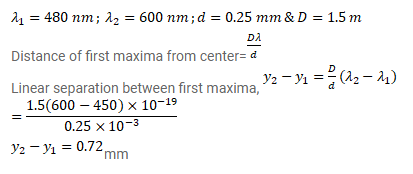# A source of light emitting light of wavelengths`
Question:

A source of light emitting light of wavelengths $480 \mathrm{~nm}$ and $600 \mathrm{~nm}$ is used in a double slit interference experiment. The separation between the slits is $0.25 \mathrm{~mm}$ and the interference is observed on a screen placed at $150 \mathrm{~cm}$ from the slits. Find the linear separation between the first maximum (next to the central maximum) corresponding to the two wavelengths.

Solution: